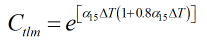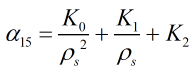# C222 – API Calculation of Ctl (Product Groups)

## Description

This calculates the correction factor to convert volumes between different temperature conditions.

Kelton calculation reference C222

This calculation is used by the following calculations:

F022/K209 – IP Paper 1 Density Referral

F023/K212 – API Density Referral

Manual of Petroleum Measurement Standards Chapter 11.1 – Volume correction factors Volume 10 – Background, Development and Program Documentation: API Standard 2540 First Edition (August 1980)

## Options

### Product Group

The constants to represent the properties of the various product groups are given in C022.

### Precision

• Rounding Select this option to follow the procedure for rounding and truncating as stated in the standard
• Full Calculate using the full precision

## Calculations

All calculations are performed in SI units unless otherwise stated

### Ctlm

The temperature correction factor is calculated bywhere:

ΔT = The difference between the temperature and the base temperature of 15 °C

andwhere K0, K1 and K2 are taken from the product group constants